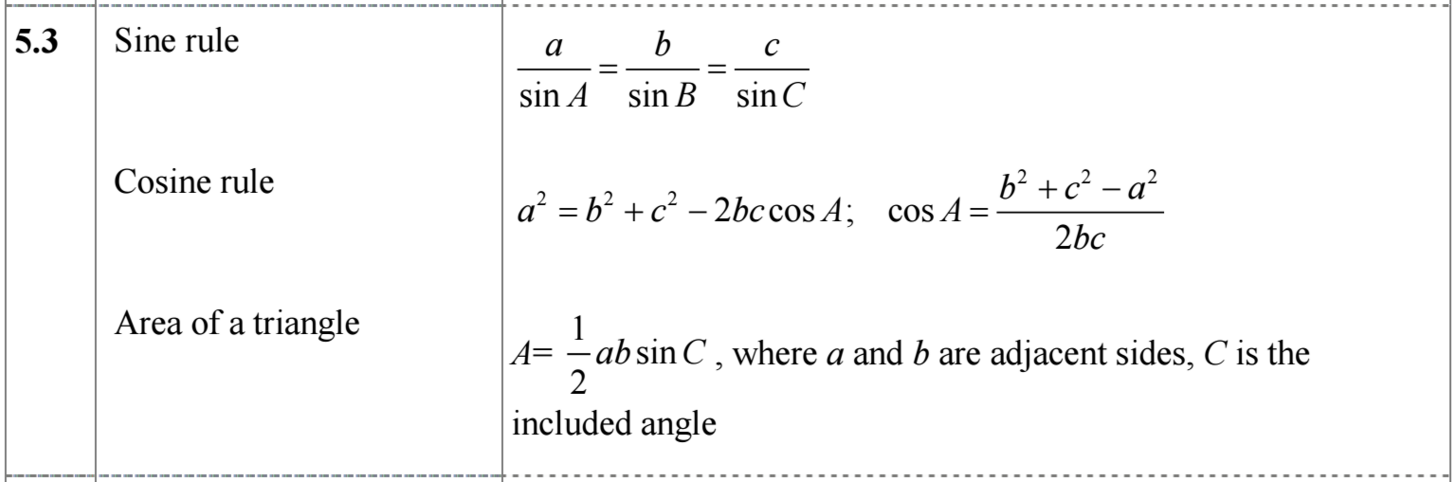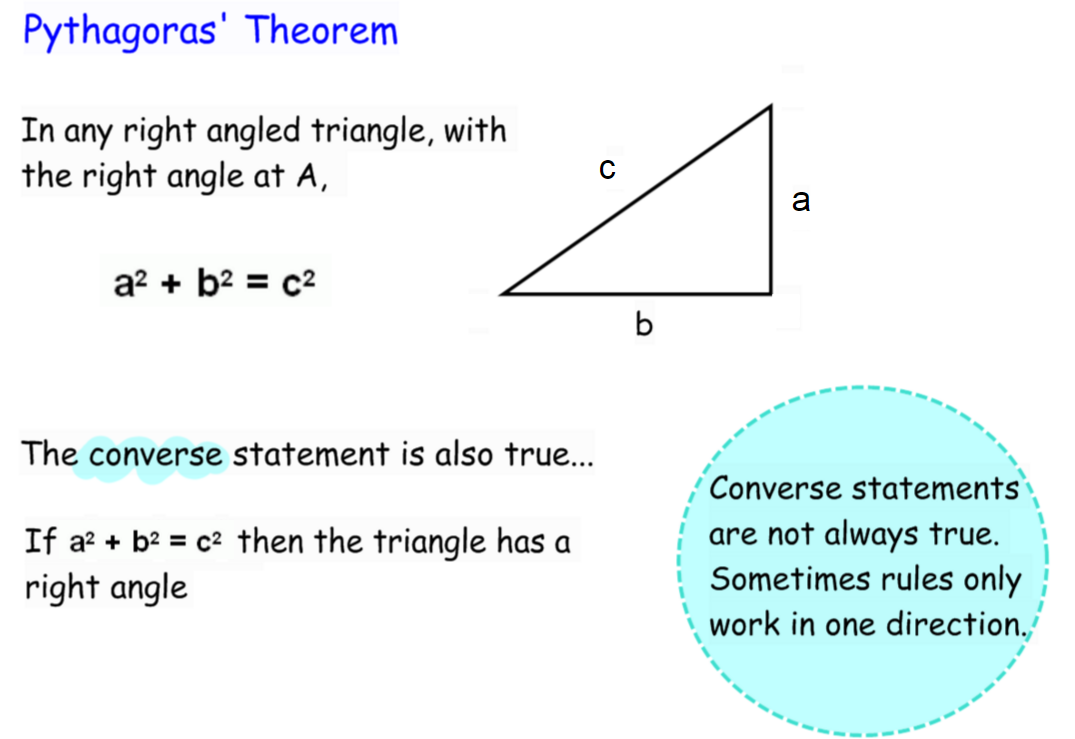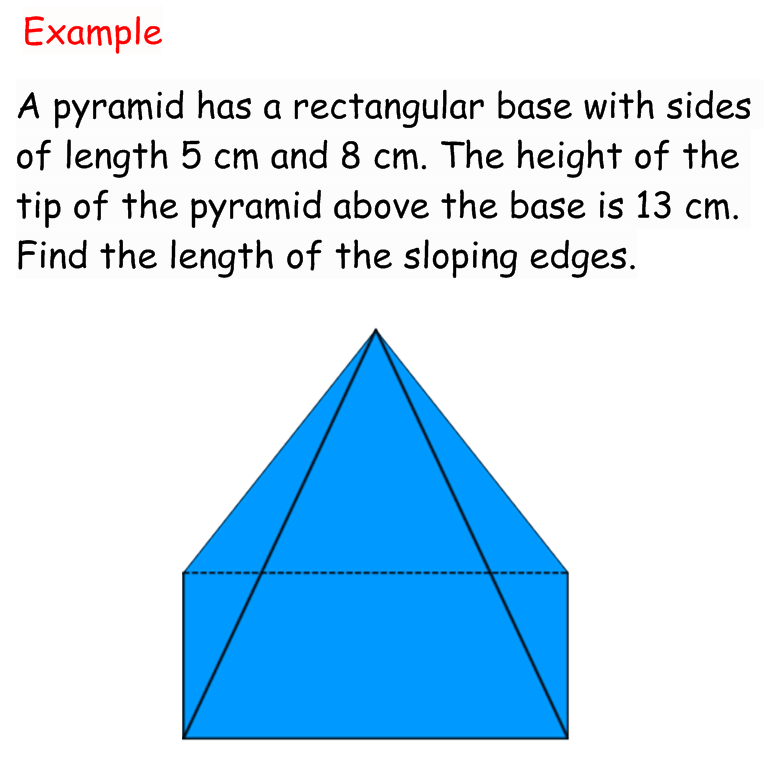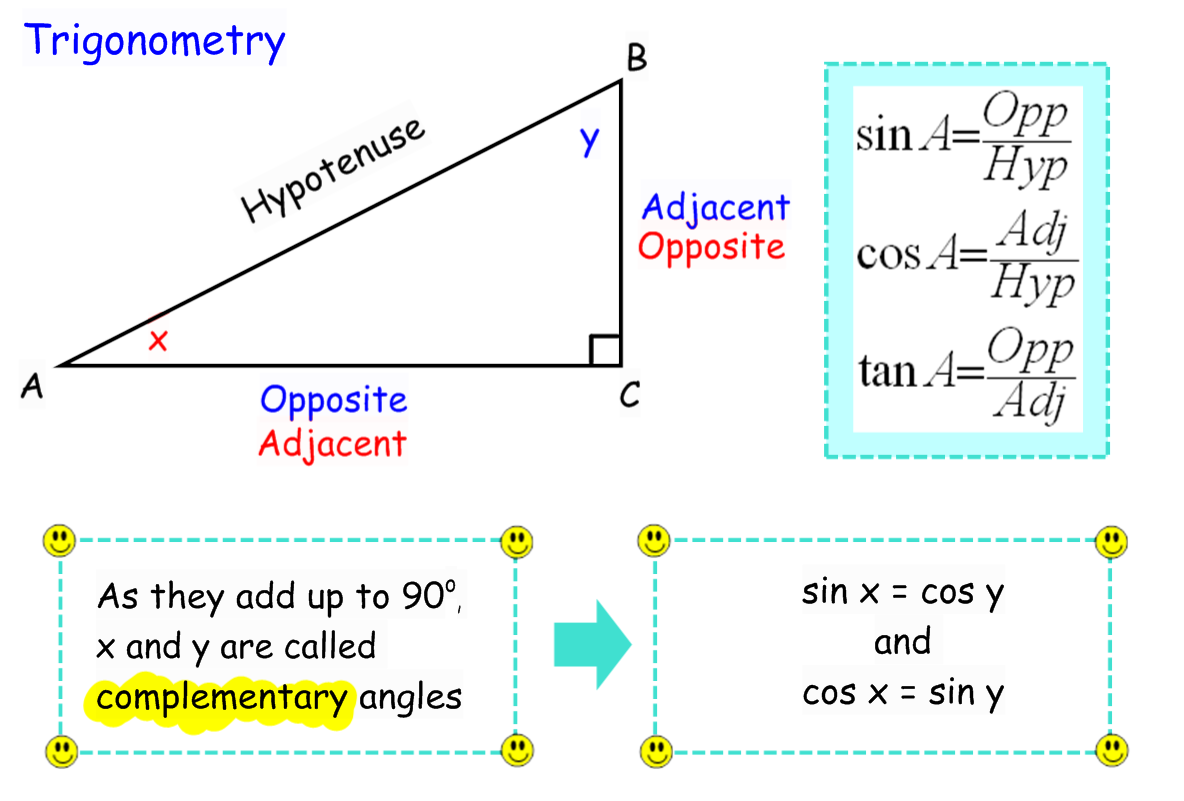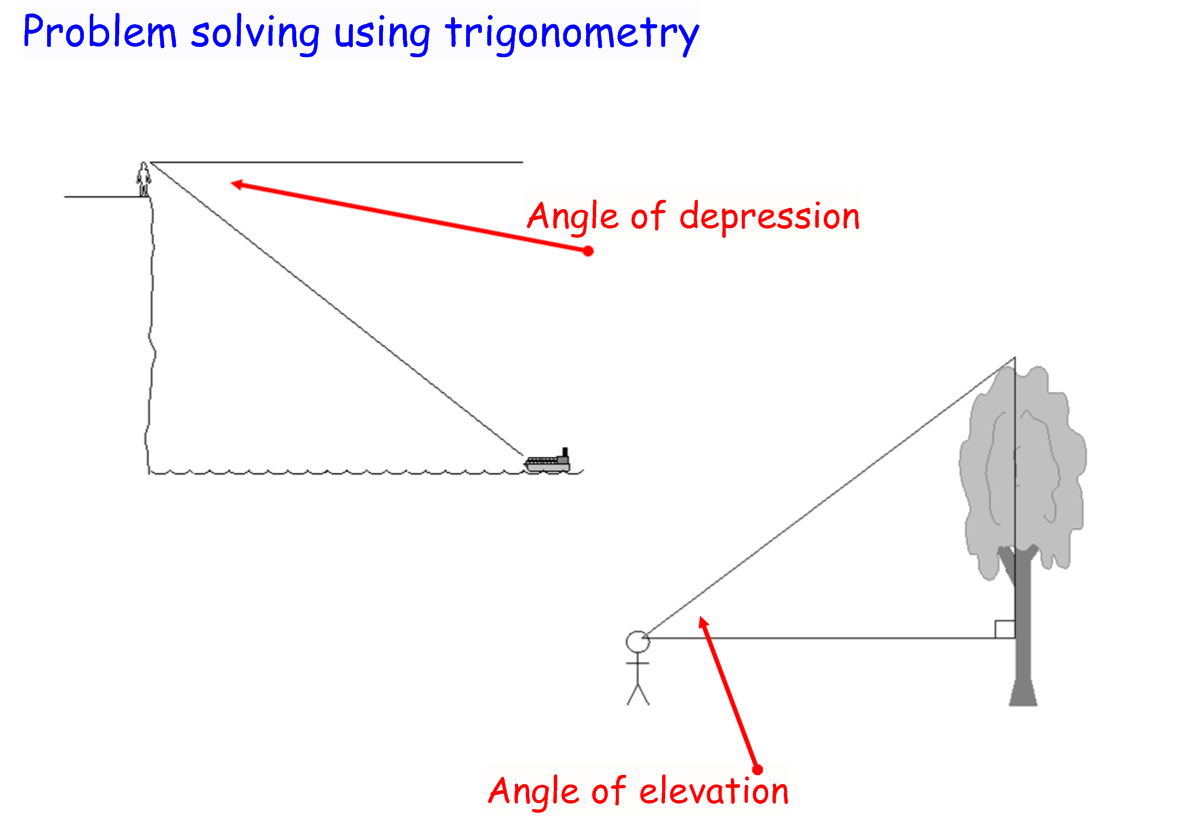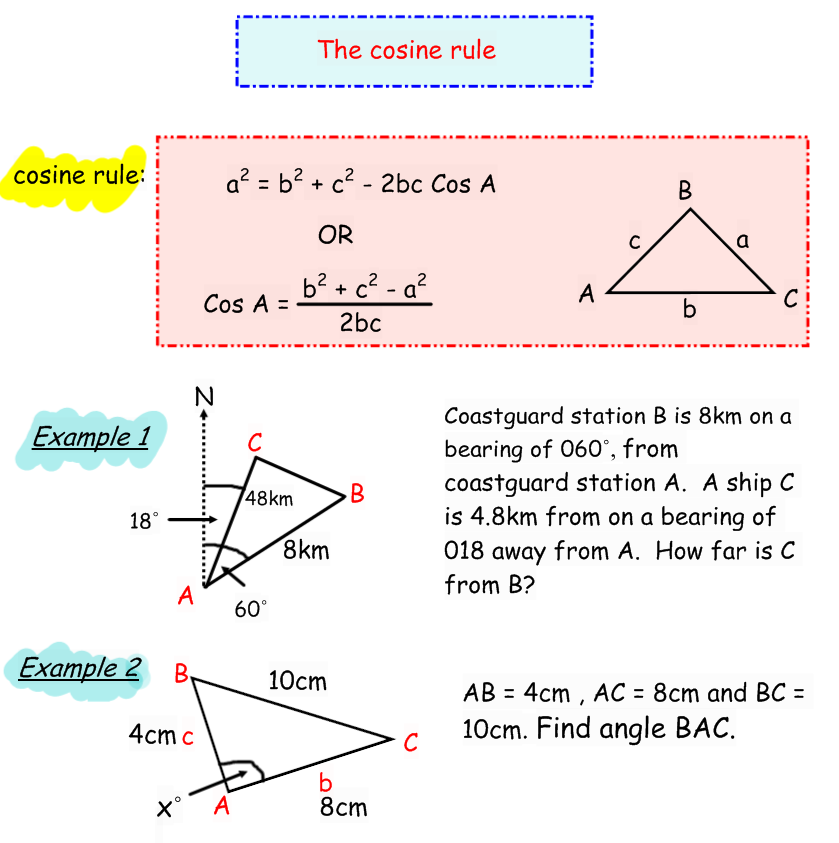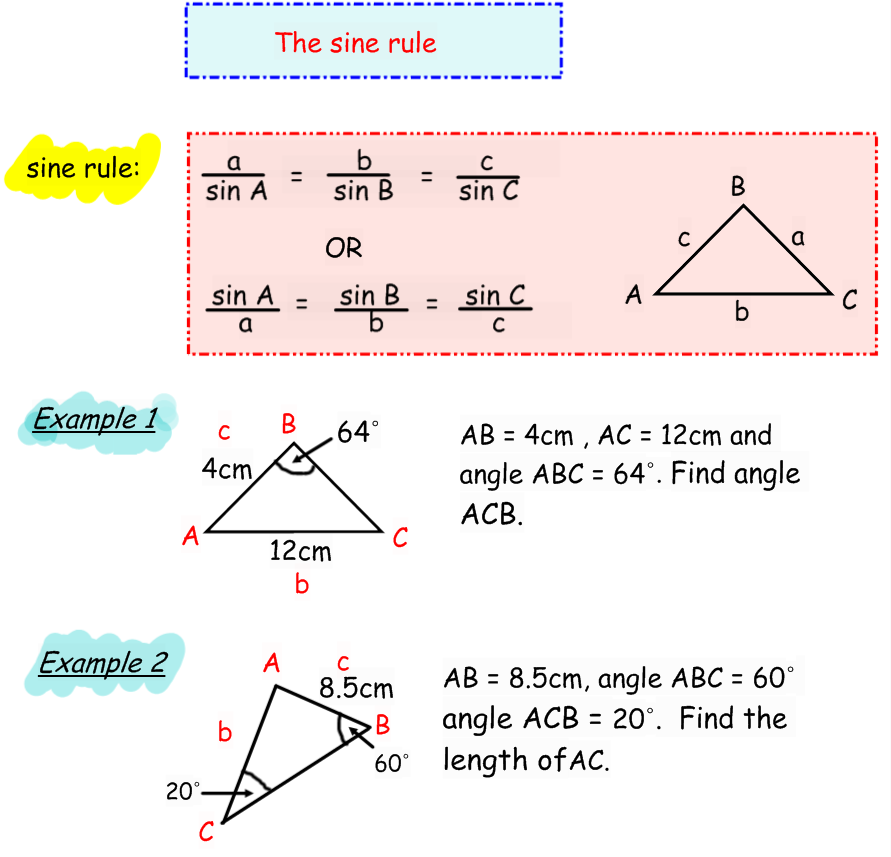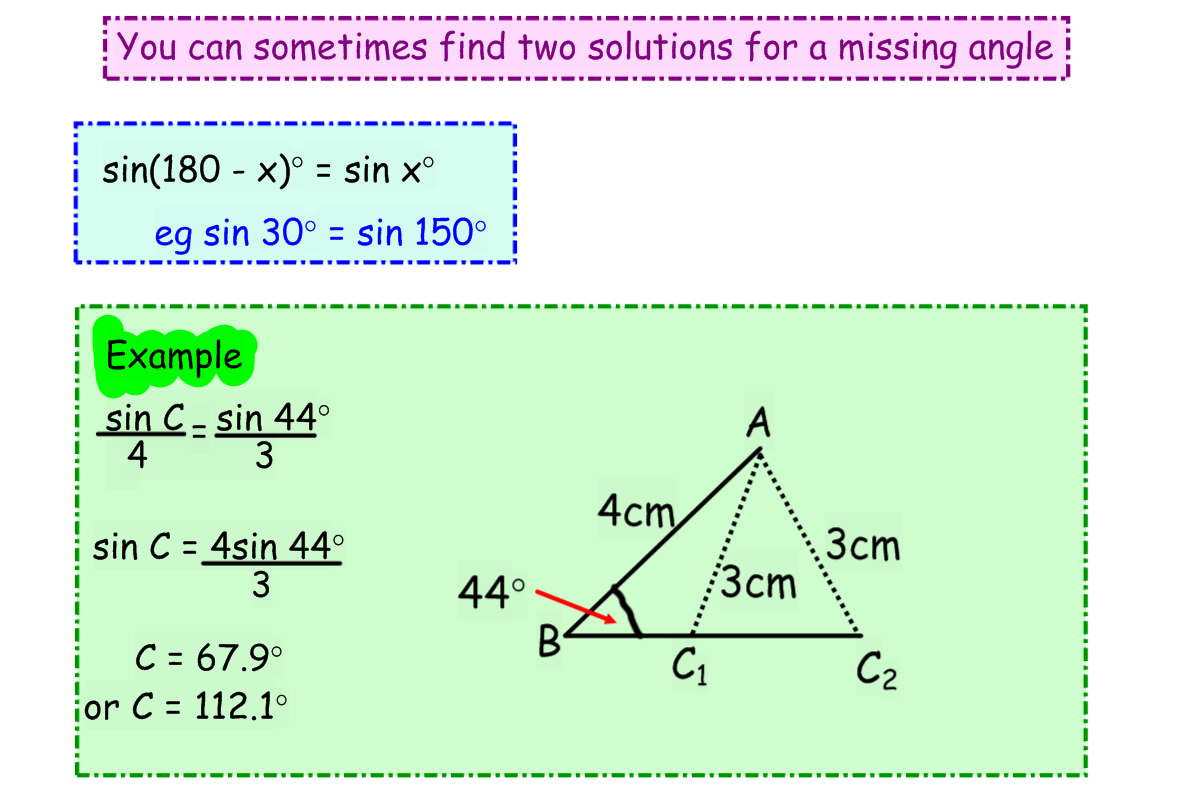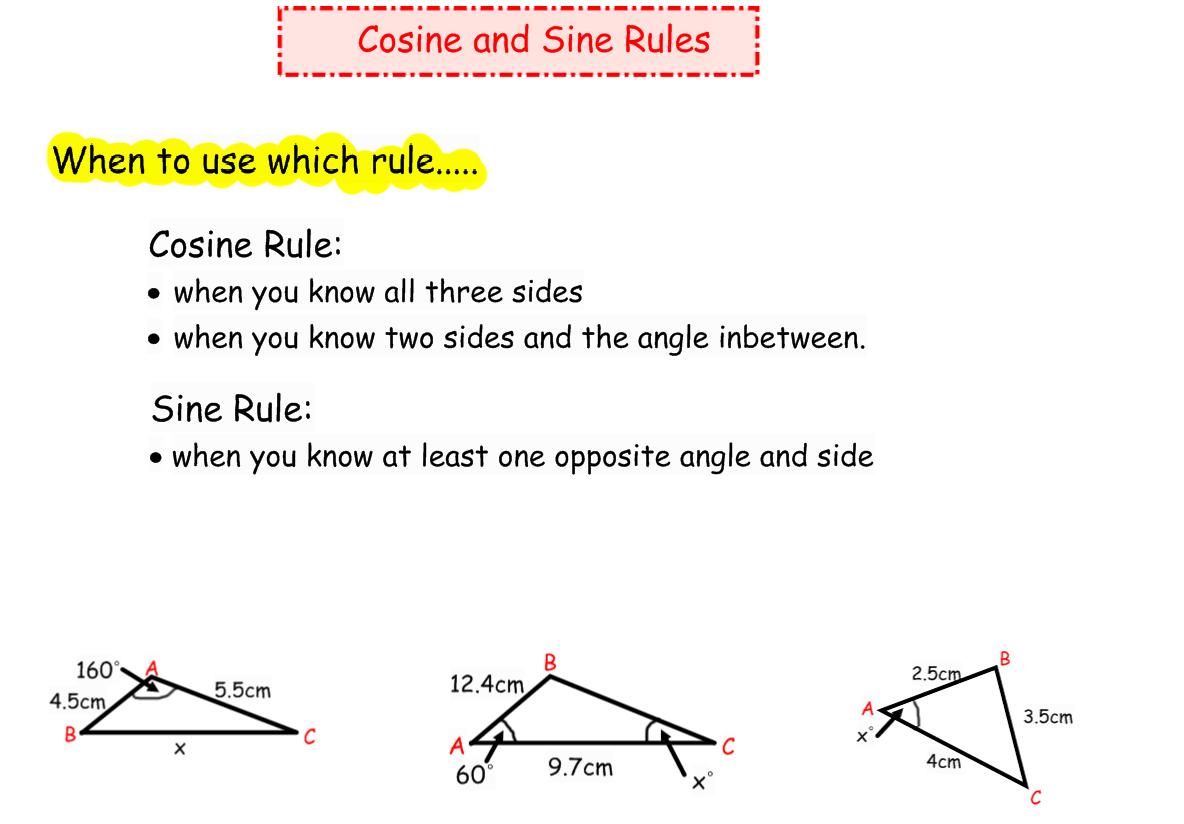### 04. Trigonometry

IBO Syllabus

Topic 5 - Geometry and Trigonometry

5.2 Use of sine, cosine and tangent ratios to find the sides and angles of a right-angled triangle; angles of depression and elevation
5.3 Use of the sine rule and the cosine rule; use of area of a triangle; construction of labeled diagrams from verbal statements
5.4 Geometry of three-dimensional solids; distance between two points; angle between two lines or between a line and a plane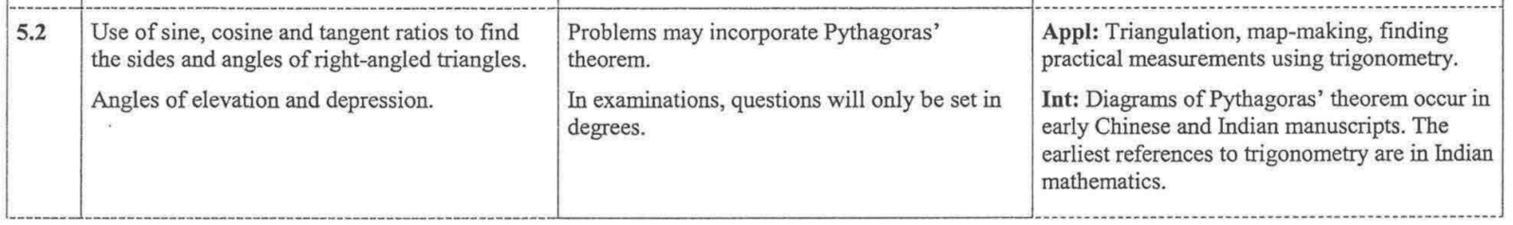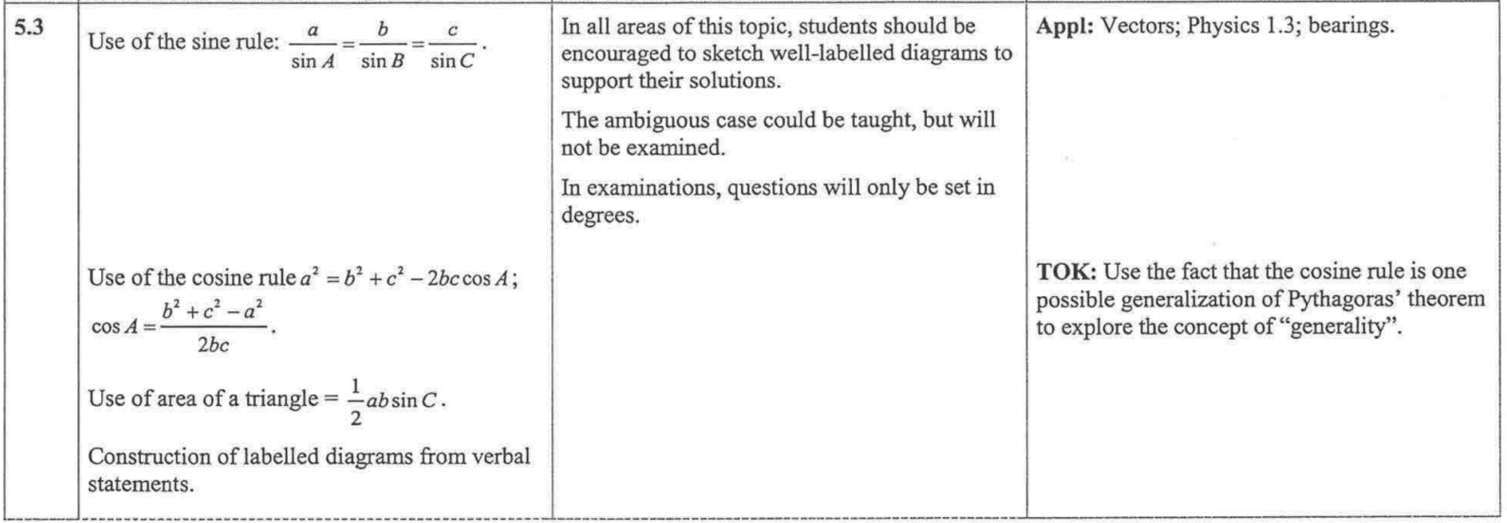Textbook Reference

Prior Learning p 533-539
3.1 Pythagoras' theorem
3.3 Two-dimensional shapes
3.4 Perimeter
3.5 Area

3.3 The sine, cosine and tangent ratios p103-119
- Finding the sides of a right-angle triangle
- Finding the angles of a right angle triangle
- Finding right-angled triangles in other shapes
- Angles of elevation and depression

3.4 The sine and coseine rules p119-125
- The sine rule
- The cosine rule
- Area of a triangle

Review exercises p126-128
Paper 1 style questions Q5-8
Paper 2 style questions Q2-3

10.2 Distance between two points in a solid p426-429

10.3 Angles between two lines, or between a line and a plane p429-436

Review exercises p448-450
Paper 1 style questions Q3-5,7
Paper 2 style questions Q1-4

IBO Formula Booklet